# Intermediate Geometry : How to find if kites are similar

## Example Questions

### Example Question #1 : How To Find If Kites Are Similar

A kite has two different side lengths ofand. Find the measurements for a similar kite.andandandandandandExplanation:

A kite is a geometric shape that has two sets of equivalent adjacent sides. In order for two kites to be similar their sides must have the same ratios.

Since, the given kite has side lengthsand, they have the ratio of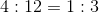.

Therefore, find the side lengths that have a ratio of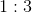.

The only answer choice with this ratio is: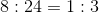### Example Question #2 : How To Find If Kites Are Similar

A kite has two different side lengths ofand. Find the measurements for a similar kite.andandandandandandExplanation:

A kite is a geometric shape that has two sets of equivalent adjacent sides. In order for two kites to be similar their sides must have the same ratios.

The given side lengths for the kite areand, which have the ratio of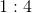The only answer choice with the same relationship between side lengths is:and, which has the ratio of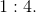### Example Question #3 : How To Find If Kites Are Similar

Suppose the ratio of a kite's side lengths isto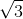.  Find a similar kite.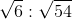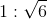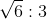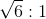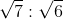Explanation:

To find a similar kite, first take the ratios of the two sides and convert this to fractional form.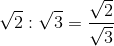Rationalize the denominator.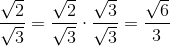The ratios of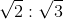matches that of.

### Example Question #4 : How To Find If Kites Are Similar

Suppose a kite has side lengths ofand.  What must the side lengths be for a similar kite?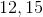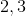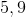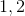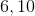Explanation:

Write the side lengths 4 and 5 as a ratio.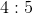The only side lengths that match this ratio by a scale factor ofis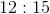.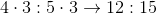Therefore, the correct side lengths are.